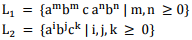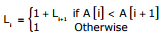# Theory of Computation - Online Test

Q1. S → a Sa| bSb| a| b The language generated by the above grammar over the alphabet {a, b} is the set of
Explaination / Solution:
No Explaination.

Q2. Which one of the following languages over the alphabet {0, 1} is described by the regular expression: (0 + 1)* 0 (0 + 1)* 0(0 + 1)*?
Explaination / Solution:
No Explaination.

Q3. Which one of the following is FALSE?
Explaination / Solution:
No Explaination.

Q4. Let L = L1 ∩ L2, where L1 and Lare languages as defined below:Then L is
Explaination / Solution:
No Explaination.

Q5. Consider two transactions T1 and T2, and four schedules S1, S2, S3, S4 of T1 and T2 as given below:Which of the above sche dules are conflict-serializable?
Explaination / Solution:
No Explaination.

Q6. If the difference between the expectation of the square of random variable (E[X2]) and the square of the expectation of the random variable (E[X2]) is denoted by R then
Explaination / Solution:
No Explaination.

Q7. The lexical analysis for a modern computer language such as Java needs the power of which one of the following machine models in a necessary and sufficient sense?
Explaination / Solution:

Lexical Analysis is implemented by finite automata

Q8. An algorithm to find the length of the longest monotonically increasing sequence of numbers in an array A[0 : n - 1] is given below.
Let Li denote the length of the longest monotonically increasing sequence starting at index i in the array
Initialize Ln-1 =1
For all i such that 0 ≤ i ≤ n − 2Finally the length of the longest monotonically increasing sequence is max (L0L1,....,Ln-1). Which of the following statements is TRUE?
Explaination / Solution:
No Explaination.

Q9. Let P be a regular language and Q be a context free language such that Q ⊆ P. (For example, let P be the language represented by the regular expression p*q* and Q be {pn qn | n ∈ N}). Then which of the following is ALWAYS regular?
Explaination / Solution:

Σ* − P is the complement of P so it is always regular, since regular languages are closed under complementation

Q10. Which of the following pairs have DIFFERENT expressive power?
Explaination / Solution:

NPDA is more powerful than DPDA.